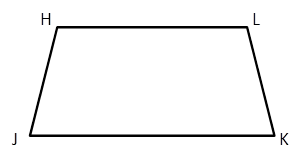Chapter 4.4, Problem 8EElementary Geometry For College St...

7th Edition
Alexander + 2 others
ISBN: 9781337614085

Solutions

Chapter
SectionElementary Geometry For College St...

7th Edition
Alexander + 2 others
ISBN: 9781337614085
Textbook Problem

If ∠ H       a n d       ∠ J are supplementary in HJKL, area) ∠ K       a n d       ∠ L necessarily supplementary also.b) ∠ H       a n d       ∠ K necessarily supplementary.To determine

a)

To explain:

The angles KandL are supplementary.

Explanation

Consider the following trapezoid HJKL.

It is given that, in a given trapezoid HandJ are supplementary.

It represents HL and JK are parallel lines and these two lines are cut by a transversal HJ and LK

To determine

b)

To explain:

The angles HandK are supplementary.

Still sussing out bartleby?

Check out a sample textbook solution.

See a sample solution

The Solution to Your Study Problems

Bartleby provides explanations to thousands of textbook problems written by our experts, many with advanced degrees!

Get Started

Absolute Value Evaluate each expression. 72. (a) |624| (b) |712127|

Precalculus: Mathematics for Calculus (Standalone Book)

Fill in each blank: 5yd=ft

Elementary Technical Mathematics

In Problems 9-20, graph each function. 10.

Mathematical Applications for the Management, Life, and Social Sciences